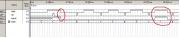Программируемая логика - это не так уж и сложно. Разберемся вместе.

Вт мар 28, 2017 21:00:00

Добрый день! Помогите пожалуйста разобраться почему происходит именно так, а не иначе.
Сразу перейду непосредственно к сути.
Вот текст очень небольшого модуля на языке Verilog:
Код:
`module filter(   input wire data,                 input wire clk,                    output reg signal,   output reg [1:0]state);always @ (posedge clk)begin   case(state)      0: state <= 1;      1: state <= 2;      2: state <= 3;      3: state <= 0;   endcase   endalways @ (state)begin   case(state)      0: signal <= signal;      1: signal <= data;      2: signal <= signal;      3: signal <= signal;   endcase   endendmodule`

Задумка была такая. При каждом фронте "clk" меняется переменная "state", а при каждом изменении "state" меняется состояние сигнала выходной переменной "signal".
При "state=1" в выходную переменную"signal" записывается значение входа "data", в остальных случаях переменная "signal" не должна менять своего значения.
По идее значение переменной "signal" должно обновляться только при изменении переменной "state", так как только она заключена в так называемый список чувствительности (always @ (state)).
Но на деле ее значение повторяет входной сигнал "data", при неизменяемой "state=1". Как будто при "state=1" между "data" и "signal" просто проводник. Прикладываю скриншот из симулятора.
Буду признателен за подсказки.Ср мар 29, 2017 22:37:00

Если кому интересно причина такого поведения следующая:
При описании выражений внутри процедурных блоков комбинаторной логики
регистры, которым идет присвоение не будут выполнены в виде D-триггеров после синтеза.
Описание синхронной логики очень похоже на описание
комбинаторной логики с одним важным отличием – в списке чувствительности
always блока указывают не сами сигналы, а фронт тактовой частоты clock. Только в этом случае регистры,
которым идет присвоение, будут реализованы в виде D-триггеров.
На всякий случай выложу рабочий модуль. Мало ли.
Код:
`module filter(   input wire data,                input wire clk,                    output reg signal,   output reg [1:0]state);always @ (posedge clk)begin   case(state)      0: begin state <= 1; signal <= data; end;      1: begin state <= 2; signal <= signal; end;      2: begin state <= 3; signal <= signal; end;      3: begin state <= 0; signal <= signal; end;   endcase   endendmodule`